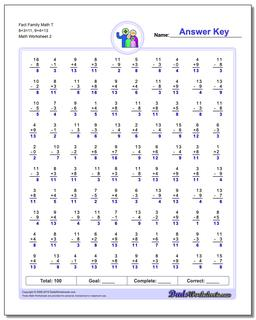# Math Worksheets: Fact Family Math: Fact Family Math: Fact Family Math T 8+3=11, 9+4=13 (Second Worksheet)## Fact Family Math T 8+3=11, 9+4=13 (Second Worksheet)

PropertyValue
DescriptionFact Family Math T 8+3=11, 9+4=13: These math worksheets have 100 addition and subtraction fact family problems and make for a challenging two minute test. (Second Worksheet)
Resource TypeWorksheet